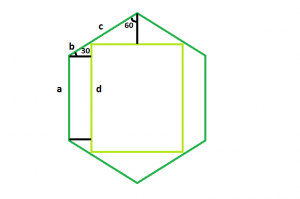Open in App
Not now

# Largest Square that can be inscribed within a hexagon

• Last Updated : 07 Aug, 2022

Given a regular hexagonof side length a, the task is to find the area of the largest square that can be inscribed within it.
Examples:

Input: a = 6
Output: 57.8817
Input: a = 8
Output: 102.901Approach: The square we will derive will have the same centre and axes of the hexagon. This is because the square will become smaller if we will rotate it.

The sides of the hexagonal are equal i.e. a = b + c
Now, let d be the length of the side of the inscribed square,
Then the top side of the square, d = 2 * c * sin(60)
And, the left side of the square, d = a + 2 * b * sin(30)
Substituting for c, d = 2 * (a – b) * sin(60)
Now taking d and re-arranging, we get, b / a = (2 * sin(60) – 1) / (2 * (sin(30) + sin(60)))
So, b / a = 2 – √3
Now, substituting the relation of b and a in the left hand side equation of square, we get,
d / a = 3 – √3 i.e. d / a = 1.268
Therefore, d = 1.268 * a

Below is the implementation of the above approach:

## C++

 `// C++ program to find the area of the largest square` `// that can be inscribed within the hexagon` `#include ` `using` `namespace` `std;`   `// Function to find the area` `// of the square` `float` `squareArea(``float` `a)` `{`   `    ``// Side cannot be negative` `    ``if` `(a < 0)` `        ``return` `-1;`   `    ``// Area of the square` `    ``float` `area = ``pow``(1.268, 2) * ``pow``(a, 2);` `    ``return` `area;` `}`   `// Driver code` `int` `main()` `{` `    ``float` `a = 6;` `    ``cout << squareArea(a) << endl;` `    ``return` `0;` `}`

## Java

 `// Java program to find the area of the largest square` `// that can be inscribed within the hexagon` `class` `Solution {` `    ``// Function to find the area` `    ``// of the square` `    ``static` `float` `squareArea(``float` `a)` `    ``{`   `        ``// Side cannot be negative` `        ``if` `(a < ``0``)` `            ``return` `-``1``;`   `        ``// Area of the square` `        ``float` `area = (``float``)(Math.pow(``1.268``, ``2``) * Math.pow(a, ``2``));` `        ``return` `area;` `    ``}`   `    ``// Driver code` `    ``public` `static` `void` `main(String args[])` `    ``{` `        ``float` `a = ``6``;` `        ``System.out.println(squareArea(a));` `    ``}` `}`   `// This code is contributed by Arnab Kundu`

## Python3

 `# Python program to find the area of the largest square` `# that can be inscribed within the hexagon`   `# Function to find the area` `# of the square` `def` `squareArea(a):`   `    ``# Side cannot be negative` `    ``if` `(a < ``0``):` `        ``return` `-``1``;`   `    ``# Area of the square` `    ``area ``=` `(``1.268` `*``*` `2``) ``*` `(a ``*``*` `2``);` `    ``return` `area;`   `# Driver code` `a ``=` `6``;` `print``(squareArea(a));`   `# This code contributed by PrinciRaj1992 `

## C#

 `// C# program to find the area of the largest square` `// that can be inscribed within the hexagon` `using` `System;` `class` `Solution {` `    ``// Function to find the area` `    ``// of the square` `    ``static` `float` `squareArea(``float` `a)` `    ``{`   `        ``// Side cannot be negative` `        ``if` `(a < 0)` `            ``return` `-1;`   `        ``// Area of the square` `        ``float` `area = (``float``)(Math.Pow(1.268, 2) * Math.Pow(a, 2));` `        ``return` `area;` `    ``}`   `    ``// Driver code` `    ``public` `static` `void` `Main()` `    ``{` `        ``float` `a = 6;` `        ``Console.WriteLine(squareArea(a));` `    ``}` `}`   `// This code is contributed by  anuj_67..`

## PHP

 ``

## Javascript

 ``

Output:

`57.8817`

Time Complexity: O(1)

Auxiliary Space: O(1)

My Personal Notes arrow_drop_up
Related Articles